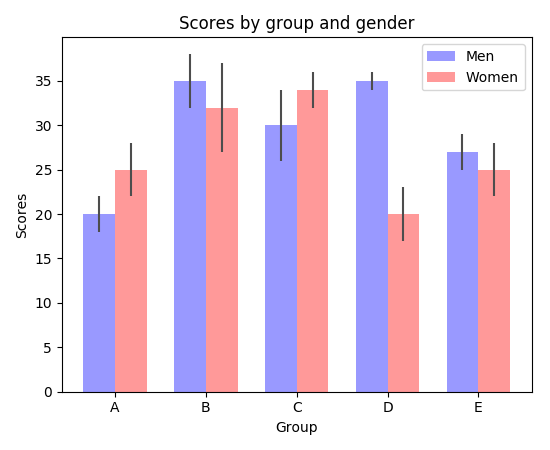Travis-CI:api example code: barchart_demo.py¶"""
========
Barchart
========

A bar plot with errorbars and height labels on individual bars
"""
import numpy as np
import matplotlib.pyplot as plt

N = 5
men_means = (20, 35, 30, 35, 27)
men_std = (2, 3, 4, 1, 2)

ind = np.arange(N)  # the x locations for the groups
width = 0.35       # the width of the bars

fig, ax = plt.subplots()
rects1 = ax.bar(ind, men_means, width, color='r', yerr=men_std)

women_means = (25, 32, 34, 20, 25)
women_std = (3, 5, 2, 3, 3)
rects2 = ax.bar(ind + width, women_means, width, color='y', yerr=women_std)

# add some text for labels, title and axes ticks
ax.set_ylabel('Scores')
ax.set_title('Scores by group and gender')
ax.set_xticks(ind + width / 2)
ax.set_xticklabels(('G1', 'G2', 'G3', 'G4', 'G5'))

ax.legend((rects1, rects2), ('Men', 'Women'))

def autolabel(rects):
"""
Attach a text label above each bar displaying its height
"""
for rect in rects:
height = rect.get_height()
ax.text(rect.get_x() + rect.get_width()/2., 1.05*height,
'%d' % int(height),
ha='center', va='bottom')

autolabel(rects1)
autolabel(rects2)

plt.show()

Keywords: python, matplotlib, pylab, example, codex (see Search examples)